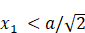1. 问题描述

2. 初始解法

2.1 三者互素的判断

3. 改进1

4. 改进2

5. 改进3

6. 测试结果

# 1. 问题描述

本题来自《程序员的算法趣题》中的第16题。

假设分别将3根长度相同的绳子摆成3个四边形。其中2根摆成长方形，另外1根摆成正方形。这时，当长度选择适当的话，会出现两个长方形的面积之和等于正方形的面积的情况（假设绳子长度和各四边形的边长都是整数）。

此外，将同比整数倍的结果看作是同一种解法。

# 2. 初始解法

令绳子长度为L=4*a，即正方形的边长为a。令另外两个长方形的较短的边分别为x1和x2，并且不失一般性可以假定它们满足关系：x1<=x2<a。

这个问题可以通过遍历搜索来解决。其中，对于任意的a，基于以上假设必然有，进一步可以推导出。这样可以将x1的搜索范围缩小。代码如下：

``````import sys
import time
import random
from math import gcd, sqrt, ceil
from   typing import List
# from   queue import Queue

class Solution:

def lines2rectangles1(self, L:int)->int:
"""
:L:    The length of the lines
:
:ret:  The number of the solutions
"""
aMax = L // 4

valid_cnt = 0
for a in range(1,aMax+1):
for x1 in range(1,ceil(a/sqrt(2))): # Assuming that x1 <= x2 < a, without loss of generality
for x2 in range(x1,a):
if x1*(2*a-x1) + x2*(2*a-x2) == a*a:
if gcd(a,x1) == 1: # gcd(a,x1) --> gcd(a,x1,x2)
valid_cnt = valid_cnt + 1
# print('a = {0}, x1 = {1}, x2 = {2}'.format(a,x1,x2))

return valid_cnt``````

## 2.1 三者互素的判断

其中，将同比整数倍的结果看作是同一种解法，这意味着，{a,x1,x2}必须满足三者互素，换句话说三者的最大公约数为1，才被计算为一个独立的答案。容易证明，在本题中，如果{a,x1,x2}满足题设要求的平方和关系的话，{a,x1,x2}三者互素等价于任何两者互素。因此，在代码中仅判断a和x1是否互素（用python中math模块中的gcd()函数）。

# 3. 改进1

在解法1中，每次条件判断都是直接计算“x1*(2*a-x1) + x2*(2*a-x2) == a*a”，这会导致非常多的重复计算。事实上针对每个a（即针对最外层的每个循环），只需要计算一次a*a；同理针对每个x1（即针对第2层的每个循环），只需要计算一次x1*(2*a-x1)。这样可以将代码优化如下：

``````    def lines2rectangles2(self, L:int)->int:
"""
:L:    The length of the lines
:
:ret:  The number of the solutions
"""
aMax = L // 4

valid_cnt = 0
for a in range(1,aMax+1): # Assuming that x1 <= x2 < a, without loss of generality
a_squ = a*a
for x1 in range(1,ceil(a/sqrt(2))):
area1 = x1*(2*a-x1)
area2 = a_squ - area1
for x2 in range(x1,a):
if x2*(2*a-x2) == area2:
if gcd(a,x1) == 1: # gcd(a,x1) --> gcd(a,x1,x2)
valid_cnt = valid_cnt + 1
# print('a = {0}, x1 = {1}, x2 = {2}'.format(a,x1,x2))
return valid_cnt``````

运行结果表明这一简单的改进导致了运行时间下降了60%！

# 4. 改进2

``````    def lines2rectangles3(self, L:int)->int:
"""
:L:    The length of the lines
:
:ret:  The number of the solutions
"""
aMax = L // 4

valid_cnt = 0
for a in range(1,aMax+1): # Assuming that x1 <= x2 < a, without loss of generality
a_squ = a*a
for x1 in range(1,ceil(a/sqrt(2))):
if gcd(a,x1) == 1: # gcd(a,x1) --> gcd(a,x1,x2)
area1 = x1*(2*a-x1)
area2 = a_squ - area1
for x2 in range(x1,a):
if x2*(2*a-x2) == area2:
valid_cnt = valid_cnt + 1
# print('a = {0}, x1 = {1}, x2 = {2}'.format(a,x1,x2))
return valid_cnt``````

运行结果表明这一简单的改进导致了运行时间进一步下降了30%！至少说明在本实现中，平方和关系的判断比互素关系的判断更为耗时。

# 5. 改进3

进一步思考可以发现，当三个四边形满足题设条件时，假设其中一个长方形（不失一般性记为长方形1）的边长分别为a-x和a+x，则长方形2的面积必然为a*a – (a-x)(a+x)=x*x。反过来，令长方形2的边长分别为a-y和a+y，可以得到长方形1的面积必然为a*a – (a-y)(a+y)=y*y，也即三者的面积（的平方根）构成一组勾股数的关系！反之，容易证明，任何满足a*2=x*2+y*2的一组数{a,x,y}都对应着满足题设条件的三个四边形。由此可知，求满足题设条件下的解等价于寻找勾股数！

``````    def lines2rectangles4(self, L:int)->int:
"""
:L:    The length of the lines
:
:ret:  The number of the solutions
"""
aMax = L // 4

valid_cnt = 0
for a in range(1,aMax+1): # Assuming that x1 <= x2 < a, without loss of generality
a_squ = a*a
for x1 in range(1,ceil(a/sqrt(2))):
if gcd(a,x1) == 1: # gcd(a,x1) --> gcd(a,x1,x2)
diff = a_squ - x1*x1
for x2 in range(ceil(a/sqrt(2)),a):
if x2*x2 == diff:
valid_cnt = valid_cnt + 1
# print('a = {0}, x1 = {1}, x2 = {2}'.format(a,x1,x2))
return valid_cnt``````

# 6. 测试结果

测试代码如下所示：

``````if __name__ == '__main__':

sln = Solution()

L = 20
tStart = time.time()
num = sln.lines2rectangles1(L)
tCost = time.time() - tStart
print('L = {0}, numSlns = {1}, tCost = {2}(sec)'.format(L,num,tCost))

L = 500
tStart = time.time()
num = sln.lines2rectangles1(L)
tCost = time.time() - tStart
print('#1: L = {0}, numSlns = {1}, tCost = {2}(sec)'.format(L,num,tCost))
tStart = time.time()
num = sln.lines2rectangles2(L)
tCost = time.time() - tStart
print('#2: L = {0}, numSlns = {1}, tCost = {2}(sec)'.format(L,num,tCost))

L = 2000
tStart = time.time()
num = sln.lines2rectangles1(L)
tCost = time.time() - tStart
print('#1: L = {0}, numSlns = {1}, tCost = {2}(sec)'.format(L,num,tCost))
tStart = time.time()
num = sln.lines2rectangles2(L)
tCost = time.time() - tStart
print('#2: L = {0}, numSlns = {1}, tCost = {2}(sec)'.format(L,num,tCost))
tStart = time.time()
num = sln.lines2rectangles3(L)
tCost = time.time() - tStart
print('#3: L = {0}, numSlns = {1}, tCost = {2}(sec)'.format(L,num,tCost))
tStart = time.time()
num = sln.lines2rectangles4(L)
tCost = time.time() - tStart
print('#4: L = {0}, numSlns = {1}, tCost = {2}(sec)'.format(L,num,tCost))             ``````

运行后得到结果：

#1: L = 2000, numSlns = 80, tCost = 4.635588645935059(sec)
#2: L = 2000, numSlns = 80, tCost = 1.9797470569610596(sec)
#3: L = 2000, numSlns = 80, tCost = 1.2058100700378418(sec)
#4: L = 2000, numSlns = 80, tCost = 0.340120792388916(sec)

最终的算法所需要的时间只有初始算法的十分之一。

上一篇：Q15: 走楼梯

下一篇：Q17：30人31足游戏

本系列总目录参见：程序员的算法趣题：详细分析和Python全解

### C语言const修饰的常量如何修改_在c语言如何修改conarry函数_gdolphinw的博客-程序员秘密

##C语言const修饰的常量如何修改。void main(){ const int cnum=10; printf("cnum=%d\n",cnum); //cnum=20; 直接修改会报错 *(int*)(&amp;cnum)=30; //可以间接的修改 printf("cnum=%d\n",cnum); }...

### 随便推点

简而言之，Windows CE其实就是一个操作系统。它是一个抢先式多任务并具有强大通信能力的Win32嵌入式操作系统，是微软专门为信息设备、移动应用、消费类电子产品、嵌入式应用等非PC领域而从头设计的战略性操作系统产品。　　 　　你也许会有一点奇怪，为什么微软会推出这个Windows CE呢？ 　　 　　不知你是否注意到，在我们的日常生活中，人们开始普遍使用手机、PDA、手持和掌上电脑等信息电器

111111

### oracle数据库内存分配（sga和pga）_cl1210930061的博客-程序员秘密

1) 原则：SGA+PGA+OS使用内存&lt;总物理RAM 2) 通过sga+pga就能大概判断系统oracle使用了多少内存了 32位版本的oracle最大支持1.75GB的SGA ...